Courses

# Past Year Questions: Impulse And Momentum Notes | EduRev

## Mechanical Engineering : Past Year Questions: Impulse And Momentum Notes | EduRev

The document Past Year Questions: Impulse And Momentum Notes | EduRev is a part of the Mechanical Engineering Course Engineering Mechanics.
All you need of Mechanical Engineering at this link: Mechanical Engineering

Question 1:A particle of unit mass is moving on a plane. Its trajectory, in polar coordinates, is given by r(t) = t2, θ(t), where f is time. The kinetic energy of the particle at time t = 2 is



Question 2:An annular disc has a mass m, inner radius R and outer radius 2R. The disc rolls on a flat surface without slipping. If the velocity of the center of mass is v, the kinetic energy of the disc is

[2014,Set-3]

Question 3:A stone with mass of 0.1 kg is catapulted as shown in the figure. The total force Fx (in N) exerted by the rubber band as a function of distance x (in m) is given by Fx = 300x2. If the store is displaced by 0.1 m from the un-stretched position (x = 0) of the rubber band, the energy stored in the rubber band is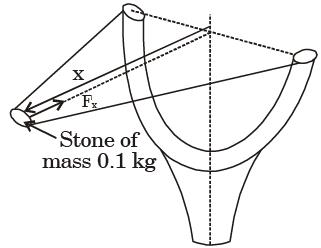Question 4:An elevator (lift) consists of the elevator cage and a counter weight, of mass m Chain each. The cage and the counter weight are connected by chain that passes over a Cage pulley. The pulley is coupled Counter weight to a motor. It is desired that the elevator should have a maximum stopping time of seconds from a peak speed v. If the inertias of the pulley and the chain are neglected, the minimum power that the motor must have isQuestion 5:An ejector mechanism consists of a helical compression spring having a spring constant of K = 981 × 103 N/m. It is pre-compressed by 100 mm from its free state. If it is used to eject a mass of 100 kg held on it, the mass will move up through a distance of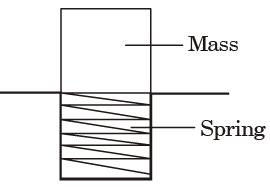Question 6:Two disc A and B with identical mass (m) and radius (R) are initially at rest. They roll down from the top of identical inclined planes without slipping. Disc A has all of its mass concentrated at the rim, while Disc B has its mass uniformly distributed. At the bottom of the plane, the ratio of velocity of the center of disc A to the velocity of the center of disc B is

Question 7:A system of particles in motion has mass center G as shown in the figure. The particle i has mass mi and its position with respect to a fixed point O is given by the position vector ri The position of the particle with respect to G is given by the vector pi, The time rate of change of the angular momentum of the system of particles about G is (The quantity pi indicates second derivative of pi with respect to time and likewise for ri).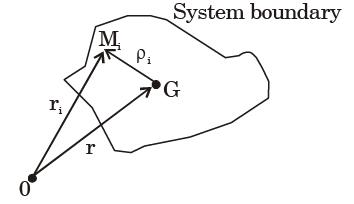Question 8:A point mass having mass M is moving with a velocity V at an angle q to the wall as shown in the figure. The mass undergoes a perfectly elastic collision with the smooth wall and rebounds. The total change (final minus initial) in the momentum of the mass is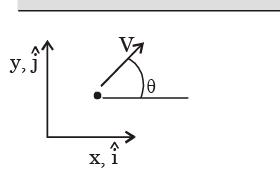Question 9:A point mass M is released from rest and slides down a spherical bowl of radius R from a height H as shown in the figure below. The surface of the bowl is smooth (no friction). The velocity of the mass at the bottom of the bowl is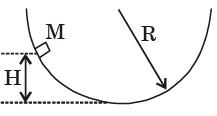[2016,Set-1]

Question 10:A mass m1 of 100 kg travelling with a uniform velocity of 5 m/s along a line collides with a stationary mass m2 of 1000 kg. After the collision, both the masses travel together with the same velocity. The coefficient of restitution is

[2014,Set-3]

Question 11:A single degree of freedom system having mass 1 kg and stiffness 10 kN/m initially at rest is subjected to an impulse force of magnitude 5 kN for 10–4 seconds. The amplitude in mm of the resulting free vibration is



Question 12:During inelastic collision of two particles, which one of the following is conserved?



Question 13:A ball of mass 3 kg moving with a velocity of 4 m/ s undergoes a perfectly-elastic directcentral impact with a stationary ball of mass m. After the impact is over, the kinetic energy of the 3 kg ball is 6 J. The possible value(s) of m is/are

[2019,Set-2]

Question 14:The coefficient of restitution of a perfectly plastic impact is



Question 15:A 1 kg mass of clay, moving with a velocity of 10 m/s, strikes a stationary wheel and sticks to it. The solid wheel has a mass of 20 kg and a radius of 1 m. Assuming that the wheel is set into pure rolling motion, the angular velocity of the wheel immediately after the impact is approximately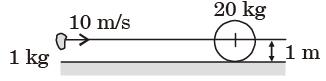Question 16:A bullet of mass m travels at a very high velocity V (as shown in the figure) and gets embedded inside the block of mass M initially at rest on a rough horizontal floor. The block with the bullet is seen to move a distance 's' along the floor.
Assuming m to be the coefficient of kinetic friction between the block and the floor and g the acceleration due to gravity. What is the velocity V of the bullet?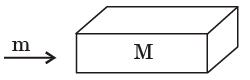Question 17:A ball A of mass m falls under gravity from a height h and strikes another ball B of mass m which is supported at rest on a spring of stiffness k. Assume perfect elastic impact. Immediately after the impact



Offer running on EduRev: Apply code STAYHOME200 to get INR 200 off on our premium plan EduRev Infinity!

## Engineering Mechanics

37 videos|85 docs|64 tests

,

,

,

,

,

,

,

,

,

,

,

,

,

,

,

,

,

,

,

,

,

;